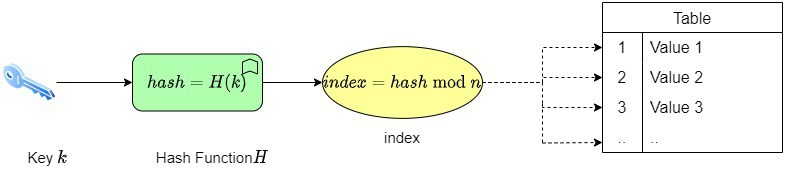## 1. Introduction

In this tutorial, we’ll study modulus division.

## 2. Euclidean Division

The division is one of the four basic arithmetic operations.

We call division with a remainder a Euclidean division. That’s the process of dividing the first number (the dividend) by the second number (the divisor) to get an integer quotient and a remainder (a natural number). The remainder is strictly lower than the divisor’s absolute value.

Formally, we can state that for any given two integersand, with, there exist unique integersandsuch that:where:is the quotient, andis the remainder.

## 3. Modulus Division

Modulus division is based on the modulus operator.

### 3.1. Modulus Operator

The modulus operator, denoted by, is an arithmetic operator that accepts two integer operandsand. Furthermore, it carries the integer division ofby, to get the remainder, which is in the interval.Furthermore, most compilers require both arguments of the modulus operator to be integers. If they aren’t, compilation reports an error.

### 3.2. Modulus Division and Clock

In this section, we relate modulus operation with the working of an analog clock.

In a typical 12-hour clock, we have 12 numbers starting with 12 at the top, 3 on the right, 6 at the bottom, and 9 on the left 12. The rest of the numbers the placed in between and the clock has three hands for hours, minutes, and seconds.

Thus, each day is divided into two 12-hour periods. Let’s say the current time is 4 pm. Now, a digital 24-hour clock will show the time as 16:00, but our analog clock will show the hour hand at 12 and the minute hand at 4. This conversion is done by the modulus operator ().

## 4. Modulus Division Applications

In this section, we look at some of the common applications of modulus division.

### 4.1. Hashing

One of the most common uses of the modulus operator is hashing. There are two steps. Firstly, we apply the hash functionto the data item key to get an integer called a hash value:Secondly, we apply the modulus division function to get the index of the hash table row that stores the original data item:Here, we have a table withrows. In a typical query, the user provides the key, and our job is to find the corresponding value:### 4.2. Find the Closest Lower Multiple of a Number

Let’s say we are given two numbersandsuch that. We want to find a numbersuch thatis the multiple ofthat is closest tobut not greater than it.

To do so, we use modulus division onandto find the remainder. Afterwards, we subtract it fromto get the nearest multiple of:For instance, ifand, we get.

## 5. Conclusion

In this article, we’ve studied division operation in general and modulus division in particular. We use the modulus division method when we need only the remainder of the integer division of the dividend and divisor. Modulus division finds huge applications in numerical methods and cryptography.